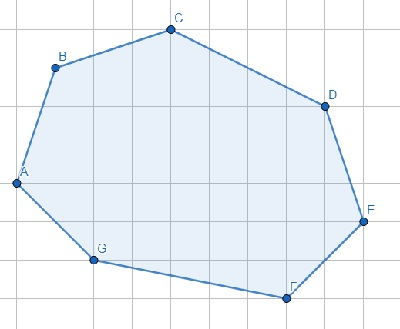# Program to check points are forming convex hull or not in Python

Suppose we have outer points of a polygon in clockwise order. We have to check these points are forming a convex hull or not.From this diagram it is clear that for each three consecutive points the interior angle is not more than 180°. So if all angles are not more than 180° then the polygon is convex hull.

So, if the input is like points = [(3,4), (4,7),(7,8),(11,6),(12,3),(10,1),(5,2)], then the output will be True.

To solve this, we will follow these steps −

• n := size of points
• for i in range 0 to size of points, do
• p1 := points[i-2] when i > 1, otherwise points[n-2]
• p2 := points[i-2] when i > 0, otherwise points[n-1]
• p3 := points[i]
• if angle between points (p1, p2, p3) > 180, then
• return False
• return True

## Example

Let us see the following implementation to get better understanding −

import math
def get_angle(a, b, c):
angle = math.degrees(math.atan2(c-b, c-b) - math.atan2(a-b, a-b))
return angle + 360 if angle < 0 else angle

def solve(points):
n = len(points)
for i in range(len(points)):
p1 = points[i-2]
p2 = points[i-1]
p3 = points[i]
if get_angle(p1, p2, p3) > 180:
return False
return True

points = [(3,4), (4,7),(7,8),(11,6),(12,3),(10,1),(5,2)]
print(solve(points))

## Input

[(3,4), (4,7),(7,8),(11,6),(12,3),(10,1),(5,2)]

## Output

True